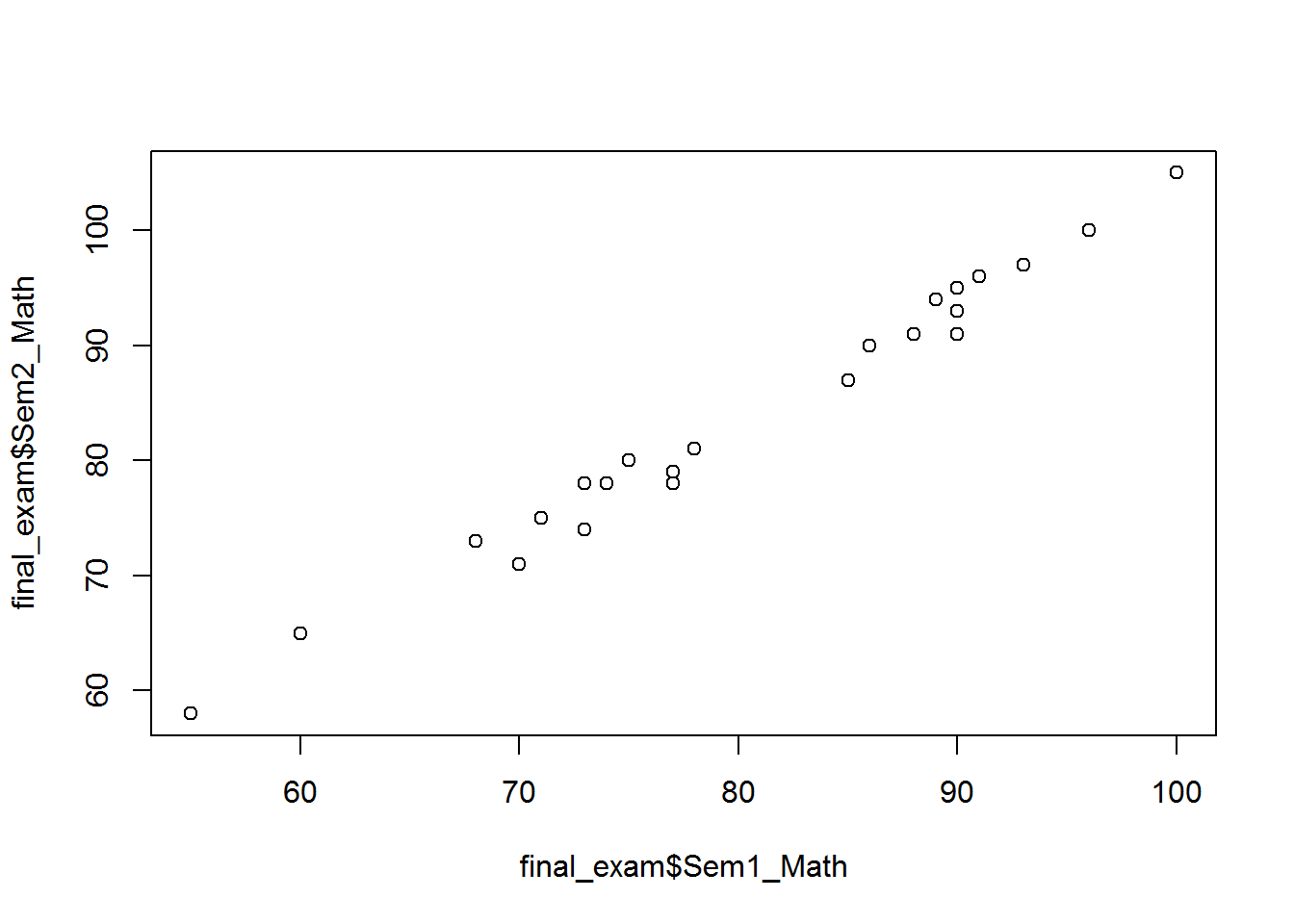• No products in the cart.

203.1.10 R Practice : Multiple Regression Issues

Practice : Multiple Regression- issues

There are some other issue in multiple regression for understanding these issue lets solve some examples. So let’s do a lab to understand other issue in building the multiple regression line. We will try to understand the problem using an example. There is final exam score data in the dataset import the final exam score data. We need to build a model that predict the final score using the rest of the variables

1. Import Final Exam Score data
2. Build a model to predict final score using the rest of the variables.
3. How are Sem2_Math & Final score related? As Sem2_Math score increases, what happens to Final score?
4. Remove “Sem1_Math” variable from the model and rebuild the model
5. Is there any change in R square or Adj R square
6. How are Sem2_Math & Final score related now? As Sem2_Math score increases, what happens to Final score?
7. Draw a scatter plot between Sem1_Math & Sem2_Math
8. Find the correlation between Sem1_Math & Sem2_Math

Solution

First let us import this final score data into the R.

1. Import Final Exam Score data

This is final exam data that has final exam marks, sem2 mathematic, sem1 mathematic, sem2 science, sem1 scienUsing four variables that are sem2 mathematic, sem1 mathematic, sem2 science, sem1 science the idea is to predict the final exam score. Create a model called exam_model, and then check the summary of the same.

1. Build a model to predict final score using the rest of the variables.
exam_model<-lm(Final_exam_marks~Sem1_Science+Sem2_Science+Sem1_Math+Sem2_Math, data=final_exam)
summary(exam_model)
##
## Call:
## lm(formula = Final_exam_marks ~ Sem1_Science + Sem2_Science +
##     Sem1_Math + Sem2_Math, data = final_exam)
##
## Residuals:
##     Min      1Q  Median      3Q     Max
## -1.7035 -0.7767 -0.1685  0.5386  3.3360
##
## Coefficients:
##              Estimate Std. Error t value Pr(>|t|)
## (Intercept)  -1.62263    1.99872  -0.812 0.426941
## Sem1_Science  0.17377    0.06281   2.767 0.012279 *
## Sem2_Science  0.27853    0.05178   5.379 3.43e-05 ***
## Sem1_Math     0.78902    0.19714   4.002 0.000762 ***
## Sem2_Math    -0.20634    0.19138  -1.078 0.294441
## ---
## Signif. codes:  0 '***' 0.001 '**' 0.01 '*' 0.05 '.' 0.1 ' ' 1
##
## Residual standard error: 1.33 on 19 degrees of freedom
## Multiple R-squared:  0.9896, Adjusted R-squared:  0.9874
## F-statistic: 452.3 on 4 and 19 DF,  p-value: < 2.2e-16
From summary it's clear that  R squared  value is  98% adjusted R squared is also 98% this means all the predicting variables that present in the model are having a good impact factor on the target variable. R-Square value of 98%, indicates that the model is a really good one for prediction
1. How are Sem2_Math & Final score related? As Sem2_Math score increases, what happens to Final score?

Sem2_Math & Final score related are inversely related. As Sem2_Math score increases Final score decreases. Let’s build a new model on the same data, we will drop sem1 mathematics from the model. Let’s use only 3 variables. The most striking difference is in the coefficient of the variable Sem2 mathematics. 4. Remove “Sem1_Math” variable from the model and rebuild the model

exam_model1<-lm(Final_exam_marks~Sem1_Science+Sem2_Science+Sem2_Math, data=final_exam)
summary(exam_model1)
##
## Call:
## lm(formula = Final_exam_marks ~ Sem1_Science + Sem2_Science +
##     Sem2_Math, data = final_exam)
##
## Residuals:
##     Min      1Q  Median      3Q     Max
## -2.2356 -1.2817  0.0549  0.8363  4.7041
##
## Coefficients:
##              Estimate Std. Error t value Pr(>|t|)
## (Intercept)  -2.39857    2.63229  -0.911 0.373037
## Sem1_Science  0.21304    0.08209   2.595 0.017302 *
## Sem2_Science  0.26859    0.06843   3.925 0.000839 ***
## Sem2_Math     0.53201    0.06737   7.897 1.42e-07 ***
## ---
## Signif. codes:  0 '***' 0.001 '**' 0.01 '*' 0.05 '.' 0.1 ' ' 1
##
## Residual standard error: 1.76 on 20 degrees of freedom
## Multiple R-squared:  0.9808, Adjusted R-squared:  0.978
## F-statistic: 341.4 on 3 and 20 DF,  p-value: < 2.2e-16

On the same dataset, this variable shows a negative coefficient earlier, but now it is showing a positive coefficient. The newly built model has good r-square value. Its accuracy hasn’t gone down

1. Is there any change in R square or Adj R square
Model R2
exam_model 0.9896 0.9874
exam_model1 0.9808 0.978

Both R2

changed slightly 6. How are Sem2_Math & Final score related now? As Sem2_Math score increases, what happens to Final score?

However Sem2_Math & Final score related are now positively related. As Sem2_Math score increases Final score also increases.

1. Draw a scatter plot between Sem1_Math & Sem2_Math
plot(final_exam$Sem1_Math,final_exam$Sem2_Math)1. Find the correlation between Sem1_Math & Sem2_Math
cor(final_exam$Sem1_Math,final_exam$Sem2_Math)
##  0.9924948

The next post is about Issue of Multicollinearity in R.

0 responses on "203.1.10 R Practice : Multiple Regression Issues"